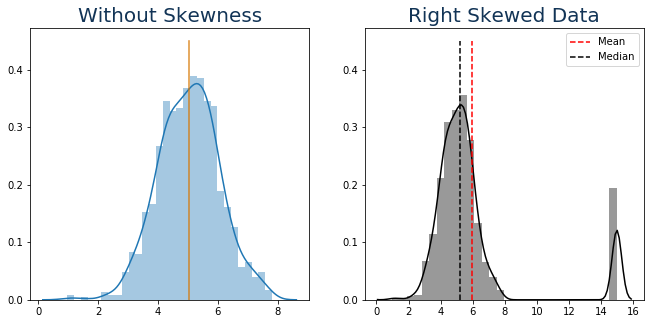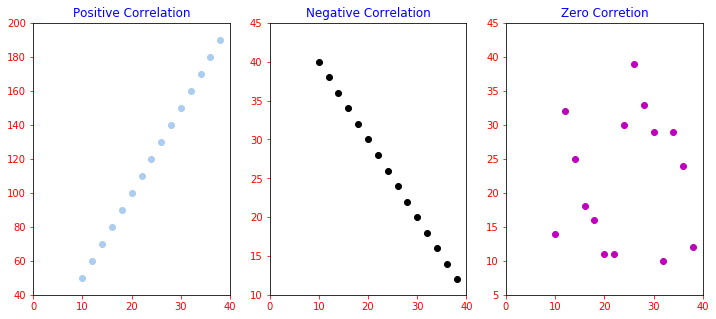•Aug 12, 2020
•Poonam
•Category: Data Science

Data science is the work of rock stars and the impact and need has only amplified with the advent of the era of Machine Learning (ML) and Big Data. There have been a lot of studies and polls done to bring about better insights into the growing popularity of data science. It has been deemed as the ‘Sexiest Job of the 21st Century’ by none other than Harvard Business Review. Not only this, Data Science has been placed on number 1 on the list of the 25 Best Job in America by Glassdoor. Data Science is the term known to most people, even laymen. And if we are to talk about the professionals, then data science is an eminent term.

The most common and sought-after of these are: Data Scientist Data Analyst Data Engineer Apart from these three highly looked upon job positions, there are many others which you can opt for. The job title you interview for depends on your interest and desire. It can be based on the work or the company and either of the reasons is fine. Here are some other job titles you can work as:

• Operations Analyst
• Systems Analyst
• Marketing Analyst
• Data Warehouse Architect
• Data Warehouse Architect
• Quantitative Analyst
• Machine Learning Engineer

Make sure you go through the most extensive questions and this is exactly what you will get in this blog. This blog contains Top 10 Data Science Interview Questions compiled by industry experts who have years of experience in the field. Each question is unique but you can be certain to learn a great deal as you move forward. The list is especially created keeping in mind the changing needs of companies and the consequent need for job seekers to change along. Well, Data Science Training and Certification course will help you to become expert in the field.

1. What is skewness??

Skewness refers to distortion or asymmetry in a symmetrical bell curve, or normal distribution, in a set of data.If the curve is shifted to the left or to the right, it is said to be skewed. Skewness can be quantified as a representation of the extent to which a given distribution varies from a normal distribution. A normal distribution has a skew of zero

There are 2 types of skewness:

1. Positive or right skewness

The mean of positively or rightskewed data will be greater than the median or mode.

2. Negative or left skewness
The mean of positively or rightskewed data will be lesser than the median or mode.
In :

```import matplotlib.pyplot as plt
import numpy as np
import seaborn as sns

ig,ax = plt.subplots(1,2,figsize=(11,5))
data = np.random.normal(5,1,1000)
mean_before = data.mean(); median_before = np.median(data)
sns.distplot(data,hist=True,ax=ax,bins=30)
ax.plot([mean_before,mean_before],[0,0.45],'r',alpha=0.5)
ax.plot([median_before,median_before],[0,0.45],'y',alpha=0.5)  #here we can see that both are equal
data= np.append(data,*100)
mean_after = data.mean()
median_after = np.median(data)
sns.distplot(data,hist=True,bins=30,color='k',ax=ax)
ax.plot([mean_after,mean_after],[0,0.45],'r--',label="Mean")
ax.plot([median_after,median_after],[0,0.45],'k--',label="Median")
ax.set_title("Right Skewed Data",fontdict={'fontsize':20,'color':'#123456'})
ax.set_title("Without Skewness",fontdict={'fontsize':20,'color':'#123456'})
plt.legend()
plt.show()In   -```
```
fig,ax = plt.subplots(1,2,figsize=(11,5))
data = np.random.normal(5,1,1000)
mean_before = data.mean(); median_before = np.median(data)
sns.distplot(data,hist=True,ax=ax,bins=30)
ax.plot([mean_before,mean_before],[0,0.45],'r',alpha=0.5)
ax.plot([median_before,median_before],[0,0.45],'y',alpha=0.5)  #here we can see that both are equal
data= np.append(data,[-0.5]*100)   #adding outliers for left skewness
mean_after = data.mean()
median_after = np.median(data)
sns.distplot(data,hist=True,bins=30,color='k',ax=ax)
ax.plot([mean_after,mean_after],[0,0.45],'r--',label='Mean')
ax.plot([median_after,median_after],[0,0.45],'k--',label='Median')
ax.set_title("Left Skewed Data",fontdict={'fontsize':20,'color':'#123456'})
ax.set_title("Without Skewness",fontdict={'fontsize':20,'color':'#123456'})
plt.legend()
plt.show()```

### 4. What is p-value and z score??

`````` In statistics p value is used for hypothesis testing. Whether to reject null hypothesis or not is depend upon the p-value.
Low p-value (≤ 0.05) indicates strength against the null hypothesis which means we can reject the null Hypothesis. High p-value (≥ 0.05) indicates strength for the null hypothesis which means we can accept the null Hypothesis p-value of 0.05 indicates the Hypothesis could go either way.

A Z-score is a numerical measurement that describes a value's relationship to the mean of a group of values. Z-score is measured in terms of standard deviations from the mean. If a Z-score is 0, it indicates that the data point's score is identical to the mean score. A Z-score of 1.0 would indicate a value that is one standard deviation from the mean. Z-scores may be positive or negative, with a positive value indicating the score is above the mean and a negative score indicating it is below the mean.``````

### 5. What is the best approach to remove the outliers from data??

``````   The best approach is to replace the outliers value with one of the following central tendency approach:
1. mean
2. median
(choose according to data)
Or we can replace with the z-score value.``````

### 6. What is the difference between overfitting , underfitting and bestfit?? Which curve to use for training model in machine learning??

``````In overfitting, a statistical model describes random error instead of the underlying relationship. Overfitting occurs when a model is excessively complex, such as having too many parameters relative to the number of observations. A model that has been overfitted, has poor predictive performance, as it overreacts to minor fluctuations in the training data.

Underfitting occurs when a statistical model or machine learning algorithm cannot capture the underlying trend of the data. Underfitting would occur, for example, when fitting a linear model to non-linear data. Such a model would have more error and less accuracy

Bestfitting occurs when a statistical model or machine learning algorithm capture the 70-80% of the underlying trend of the data and does not overreact with minor fluctuations as it cover more data while training and can easily predicto test with new observation. This fit have less error and more accuracy.

While training the model best fit curve is used``````

### 7. What is difference between cluster and classification?? What is cluster sampling??

``````classification uses predefined classes in which objects are assigned, while clustering identifies similarities between objects, which it groups according to those characteristics in common and which differentiate them from other groups of objects. These groups are known as "clusters".

Cluster sampling is a technique used when it becomes difficult to study the target population spread across a wide area and simple random sampling cannot be applied. Cluster Sample is a probability sample where each sampling unit is a collection or cluster of elements.

For eg., A researcher wants to survey the college students performanece in India. He can divide the entire population of India into different clusters (cities). Then the researcher selects a number of clusters depending on his research through simple or systematic random sampling.

Sample is small part of whole population which is used for testing.``````

### 8. Regression algorithms are best for which data type???

`````` Linear and Polynomial Regression work more accurately with the following quantitive data:
1. continuous
2. descrete

But the model results in predicted continuous value

While the logistic regression work more accurately with categorial data

example of categorial data like fail and pass, boy and girl, yes and no, types of flowers etc.

Both types of regression comes into SUPER
```

```

### 9. What is ‘Naive’ in a Naive Bayes?

``````The Naive Bayes Algorithm is based on the Bayes Theorem. Bayes’ theorem describes the probability of an event, based on prior knowledge of conditions that might be related to the event.

The Algorithm is 'naive' because it makes assumptions that may or may not turn out to be correct.``````

### 10. What is metrices in machine learning??

``````Different performance metrics are used to evaluate different Machine Learning Algorithms.
Evaluation is used to find how much error is in model and how much accurate the model is working.
We can use performance metrices like r2_score, mean_squared_error, accuracy_score, mean_absolute_error, confusion_matrix etc.``````
```
```